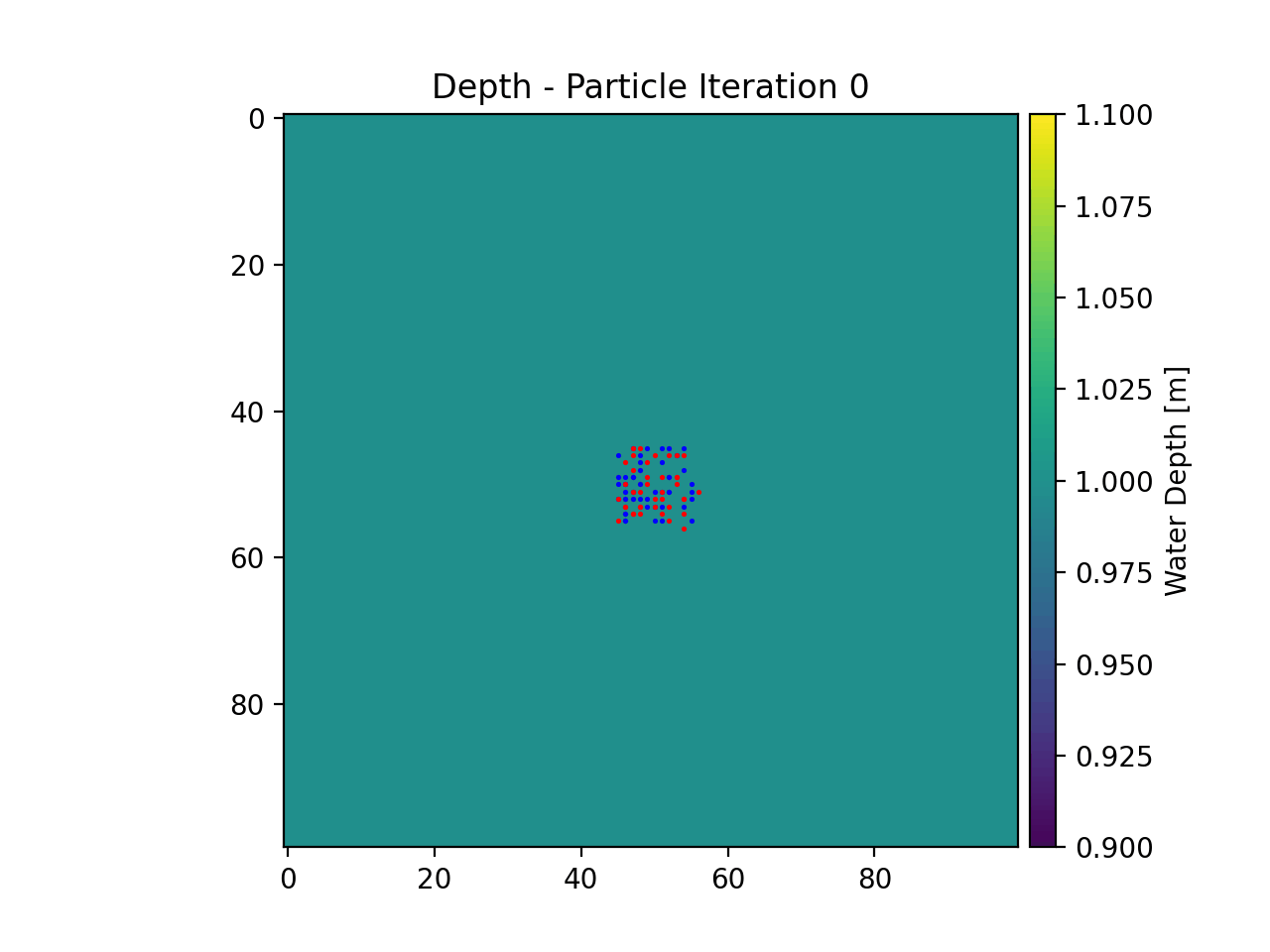# Example 9 - True Random Walk¶

dorado is fundamentally built on the concept of random walks. We can strip away the directed/weighted nature of the particle routing to return to a regular 2-D random walk process. This is what we will do in this example.

Full example script available `here`.

First we will define our parameters for the unweighted random walk. To do this, we will simulate a constant water depth, and provide no flow information. Then we will set all of our weighting parameters to 0.

```>>> import numpy as np

>>> params = particle_track.modelParams()
>>> params.depth = np.ones((100, 100))
>>> params.stage = np.ones((100, 100))
>>> params.qx = np.zeros_like(params.depth)
>>> params.qy = np.zeros_like(params.depth)
>>> params.theta = 0.0
>>> params.gamma = 0.0
>>> params.dx = 50.
>>> params.model = 'None'
```

We will initialize the `dorado.particle_track.Particles` class, seed 50 particles in the center of our artificial domain, and then simulate their movement using the steady_plots routine.

```>>> seed_xloc = list(range(45, 56))
>>> seed_yloc = list(range(45, 56))
>>> Np_tracer = 50

>>> particles = particle_track.Particles(params)
>>> particles.generate_particles(Np_tracer, seed_xloc, seed_yloc)

>>> walk_data = routines.steady_plots(particles, 50, 'true_random_walk')
```

If we animate the particle travel information we will get the below plot.